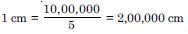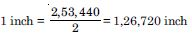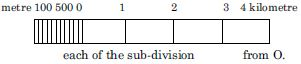# NCERT Solutions for Class 11 Geography Chapter 18 - Map Scale

##### Question 1:

Which one of the following methods of scale is a universal method?

1. Simple Statement
2. Representative Fraction
3. Graphical Scale
4. None of the above

(b). Representative Fraction

##### Question 2:

Map distance in a scale is also known as :

1. Numerator
2. Denominator
3. Statement of Scale
4. Representative Fraction

(a). Numerator

##### Question 3:

‘Numerator’ in scale represents:

1. Ground distance
2. Map distance
3. Both the distance
4. None of the above

(b). Map distance

##### Question 4:

What are the two different systems of measurement?

Metric and British.

##### Question 5:

Give one example each of statement of scale in Metric and English system.

1 metre : 100 cms, 1'' : 6 inch.

##### Question 6:

Why is the Representative Fraction method called a Universal method?

It can be used in any measurement system.

##### Question 7:

What are the major advantages of the graphical method?

It can be used is measure distance on a scale.

##### Question 8:

Convert the given statement of scale into representative fraction (RF) : 5 cm represents 10 km.

1 kilometre = 1,00,000 cm.
∴ 10 km = 10,00,000 cm
So, 5 cm = 10,00,000 cm (10 km = 10,00,000 cm)So, 1 cm = 2,00,000 cm
R.f = 1:2,00,000

##### Question 9:

Convert the given statement of scale into representative fraction (RF) : 2 inch represents 1 mile

1 mile = 63,360 inch
∴ 4 mile = 63,360 × 4 = 2,53,440 inch
So, 2 inch = 2,53,440 inchRef. = 1:1,26,720

##### Question 10:

Convert the given statement of scale into representative fraction (RF) : 1 inch represents 1 yard

1 yard = 36 inch R.F = 1:36

##### Question 11:

Convert the given statement of scale into representative fraction (RF) : 1 cm represents 100 metres

1 cm = 10,000 cm
∴ 1 metre = 10,000 cm
R : F = 1:10,000

##### Question 12:

Convert the given R.F into the statement of scale in the system of measurements shown in parenthese : 1:100,000 (into km)

1 cm, represents 1 km. Because, 1 km = 1,00,000 cm

##### Question 13:

Convert the given R.F into the statement of scale in the system of measurements shown in parenthese : 1:31,680 (into furlongs)

1 cm, represents 1 km. Because, 1 km = 1,00,000 cm

##### Question 14:

Convert the given R.F into the statement of scale in the system of measurements shown in parenthese : 1:31,680 (into furlongs)

1 inch represents 4 furlongs Because, 1 furlong–7, 920 inch So 31,680 inch = 4 furlong.

##### Question 15:

Convert the given R.F into the statement of scale in the system of measurements shown in parenthese : 1:126,720 (into miles)

1 inch represents 2 mile Because, 1 mile = 63,360 inch So, 1,26,726 inch = 2 mile

##### Question 16:

Convert the given R.F into the statement of scale in the system of measurements shown in parenthese : 1:50,000 (into metres).

1 cm represents 500 metre Because 1 mitre = 100 cm So, 50,000 cm = 500 metre Because = 1 km = 1,00,000 cm.

##### Question 17:

Construct a graphical scale when the given R.F is 1:50,000 and react the distances in kilometre and metre.